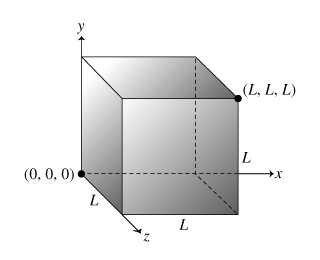# Problem: A cube has one corner at the origin and the opposite cornerat the point (L,L,L). The sides of the cube are parallel to the coordinate planes. The electric field in and around the cube is given by E = (a + bx) x̂ + c ŷ.Find the total electric flux ΦE through the surface of the cube. Express your answer in terms of a,b,c and L. What is the net charge q inside the cube? Express your answer in terms of a, b, c, L, and ϵ0.

###### FREE Expert Solution

Electric flux,

$\overline{){{\mathbf{\Phi }}}_{{\mathbf{E}}}{\mathbf{=}}\stackrel{\mathbf{⇀}}{\mathbf{E}}{\mathbf{·}}\stackrel{\mathbf{⇀}}{\mathbf{A}}}$

Gauss' law:

$\overline{){{\mathbf{\Phi }}}_{{\mathbf{E}}}{\mathbf{=}}\frac{{\mathbf{q}}_{\mathbf{e}\mathbf{n}\mathbf{c}}}{{\mathbit{ϵ}}_{\mathbf{0}}}}$

(a)

Flux through right side

x = L

E = (a + bL) + c

Aright = L2

ΦE,right = ((a + bL) + c )•(L2 ) = (a + bL)(L2 + 0) = aL2 + bL3

Flux through left side,

x = 0 - origin

E = (a + b•0) + c

Aleft = −L2

ΦE,letf = ((a + b•0)   + c )•(−L2 ) = (a)(L2 + 0) = - aL2

Flux through front side,

x = L

E = (a + bL)   + c

Afront = L2

ΦE,front = ((a + bL) + c )•(L2 ) = (0 + 0 + 0) = 0###### Problem Details

A cube has one corner at the origin and the opposite cornerat the point (L,L,L). The sides of the cube are parallel to the coordinate planes. The electric field in and around the cube is given by E = (a + bx) + c .Find the total electric flux ΦE through the surface of the cube. Express your answer in terms of a,b,c and L.

What is the net charge q inside the cube? Express your answer in terms of a, b, c, L, and ϵ0.